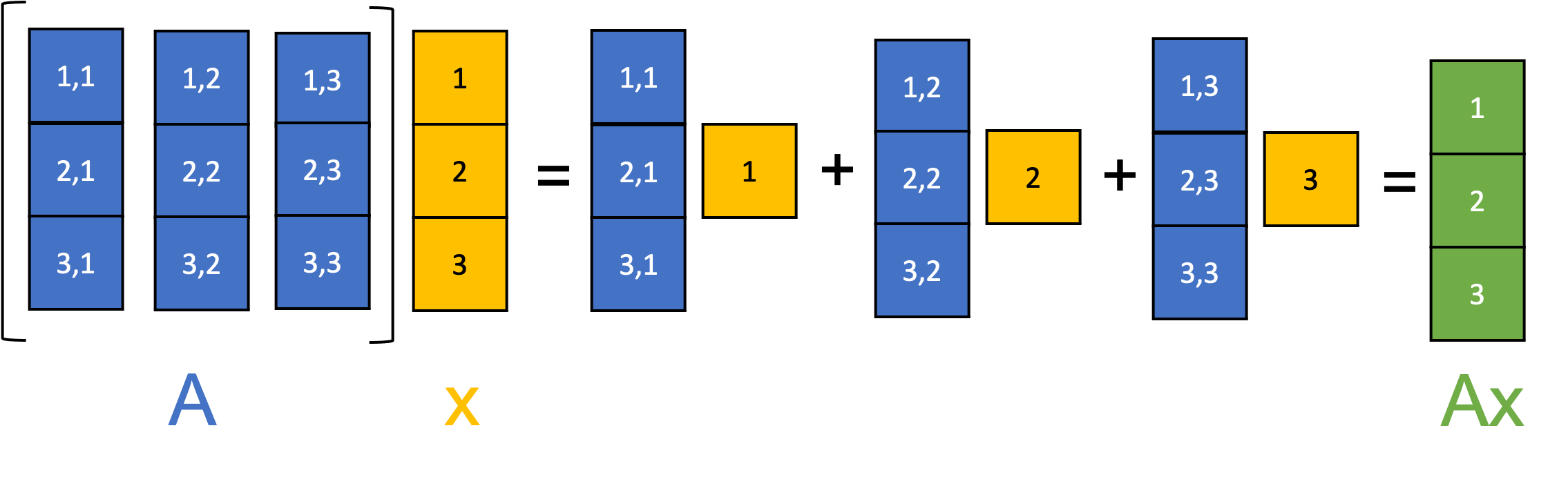# Reasoning about systems of linear equations using linear algebra

Published:

In this blog post, we will discuss the relationship between matrices and systems of linear equations. Specifically, we will show how systems of linear equations can be represented as a single matrix equation. Solutions to the system of linear equations can be reasoned about by examining the characteristics of the matrices and vectors in that matrix equation.

## Introduction

In this blog post, we will discuss the relationship between matrices and systems of linear equations. Specifically, we will show how systems of linear equations can be represented as a single matrix equation. Solutions to the system of linear equations can be reasoned about by examining the characteristics of the matrices and vectors involved in that matrix equation.

## Systems of linear equations

A system of linear equations is a set of linear equations that all utilize the same set of variables, but each equation differs by the coefficients that multiply those variables.

For example, say we have three variables, $x_1, x_2$, and $x_3$. A system of linear equations involving these three variables can be written as:

\begin{align*}3 x_1 + 2 x_2 - x_3 &= 1 \\ 2 x_1 + -2 x_2 + 4 x_3 &= -2 \\ -x_1 + 0.5 x_2 + - x_3 &= 0 \end{align*}

A solution to this system of linear equations is an assignment to the variables $x_1, x_2, x_3$, such that all of the equations are simultaneously true. In our case, a solution would be given by $(x_1, x_2, x_3) = (1, -2, -2)$.

More abstractly, we could write a system of linear equations as

\begin{align*}a_{1,1}x_1 + a_{1,2}x_2 + a_{1,3}x_3 &= b_1 \\ a_{2,1}x_1 + a_{2,2}x_2 + a_{2,3}x_3 &= b_2 \\ a_{3,1}x_1 + a_{3,2}x_2 + a_{3,3}x_3 &= b_3 \end{align*}

where $a_{1,1}, \dots, a_{3,3}$ are the coefficients and $b_1, b_2,$ and $b_3$ are the constant terms, all treated as fixed. By “fixed”, we mean that we assume that $a_{1,1}, \dots, a_{3,3}$ and $b_1, b_2,$ and $b_3$ are known. In contrast, $x_1, x_2,$ and $x_3$ are unknown. We can try different values for $x_1, x_2,$ and $x_3$ and test whether or not that assignment is a solution to the system.

## Reasoning about the solutions to a system of linear equations by respresenting the system as a matrix equation

Now, a natural question is: given a system of linear equations, how many solutions does it have? Will a system always have a solution? If did does have a solution will it only be one solution? We will use concepts from linear algebra to address these questions.

First, note that we can write a system of linear equations much more succinctly using matrix-vector multiplication. That is,

$\begin{bmatrix}a_{1,1} && a_{1,2} && a_{1,3} \\ a_{2,1} && a_{2,2} && a_{2,3} \\ a_{3,1} && a_{3,2} && a_{3,3} \end{bmatrix} \begin{bmatrix}x_1 \\ x_2 \\ x_3\end{bmatrix} = \begin{bmatrix}b_1 \\ b_2 \\ b_3\end{bmatrix}$

If we let the matrix of coefficients be $\boldsymbol{A}$, the vector of variables be $\boldsymbol{x}$, and the vector of constants be $\boldsymbol{b}$, then we could write this even more succinctly as:

$\boldsymbol{Ax} = \boldsymbol{b}$

This is an important point: any system of linear equations can be written succintly as an equation using matrix-vector multiplication. By viewing systems of linear equations through this lense, we can reason about the number of solutions to a system of linear equations using properties of the matrix $\boldsymbol{A}$!

Given this newfound representation for systems of linear equations, recall from our discussion of matrix-vector multiplication, a matrix $\boldsymbol{A}$ multiplying a vector $\boldsymbol{x}$ can be understood as taking a linear combination of the column vectors of $\boldsymbol{A}$ using the elements of $\boldsymbol{x}$ as the coefficients:Thus, we see that the solution to a system of linear equations, $\boldsymbol{x}$, is any set of weights for which, if we take a weighted sum of the columns of $\boldsymbol{A}$, we get the vector $\boldsymbol{b}$. That is, $\boldsymbol{b}$ is a vector that lies in the span of the columns of $\boldsymbol{A}$!

From this observation, we can begin to draw some conclusions about the number of solutions a given system of linear equations will have based on the properties of $\boldsymbol{A}$. Specifically, the system will either have:

1. No solution. This will occur if $\boldsymbol{b}$ lies outside the span of the columns of $\boldsymbol{A}$. This means that there is no way to construct $\boldsymbol{b}$ from the columns of $\boldsymbol{A}$, and thus there is no weights-vector $\boldsymbol{x}$ that will satisfy the equation $\boldsymbol{Ax} = \boldsymbol{b}$. Note, this can only occur if $\boldsymbol{A}$ is singular. Why? Recall that an invertible matrix maps each inpu vector $\boldsymbol{x}$ to a unique output vector $\boldsymbol{b}$ and each output $\boldsymbol{b}$ corresponds to a unique input $\boldsymbol{x}$. Said more succintly, an invertible matrix characterizes a one-to-one and onto linear tranformation. Therefore, if $\boldsymbol{A}$ is invertible, then for any given $\boldsymbol{b}$, there must exist a vector, $\boldsymbol{x}$, that solves the equation $\boldsymbol{Ax} = \boldsymbol{b}$. If such a vector does not exist, then $\boldsymbol{A}$ must not be invertible.
2. Exactly one solution. This will occur if $\boldsymbol{A}$ is invertible. As discussed above, an invertible matrix characterizes a one-to-one and onto linear transformation and thus, for any given $\boldsymbol{b}$, there will be exactly one vector, $\boldsymbol{x}$, that solves the equation $\boldsymbol{Ax} = \boldsymbol{b}$.
3. Infinitely many solutions. This will occur if $\boldsymbol{b}$ lies inside the span of the columns of $\boldsymbol{A}$, but $\boldsymbol{A}$ is not invertible. Why would there be an infinite number of solutions? Recall that if $\boldsymbol{A}$ is not invertible, then the columns of $\boldsymbol{A}$ are linearly dependent, meaning that there are an infinite number of ways to take a weighted sum of the columns of $\boldsymbol{A}$ to get $\boldsymbol{b}$. Thus, an infinite number of vectors that satisfy $\boldsymbol{Ax} = \boldsymbol{b}$.

Tags: